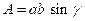Chapter 11.4, Problem 41EElementary Geometry For College St...

7th Edition
Alexander + 2 others
ISBN: 9781337614085

Solutions

Chapter
SectionElementary Geometry For College St...

7th Edition
Alexander + 2 others
ISBN: 9781337614085
Textbook Problem

Explain why the area of the parallelogram shown is given by the formula(HINT: You will need to use Q N ¯ .)Exercises 41 − 44

To determine

To explain:

The area of the Parallelogram is given by the formula A=absinγ

Explanation

Given,

From the figure,

MN¯=QP¯=b, MQ¯=NP¯=a and M=P=γ

The area of the Parallelogram(A)=The area of the triangle MNQ(A1)+ The area of the triangle QNP(A2)  The area of the triangle MNQ (A1):

For any triangle,

Here, MN¯=b, MQ¯=a and M=γ

The area of the triangle A1=12absinγ

The area of the triangle QNP (A1):

For any triangle,

Still sussing out bartleby?

Check out a sample textbook solution.

See a sample solution

The Solution to Your Study Problems

Bartleby provides explanations to thousands of textbook problems written by our experts, many with advanced degrees!

Get Started

Find more solutions based on key concepts